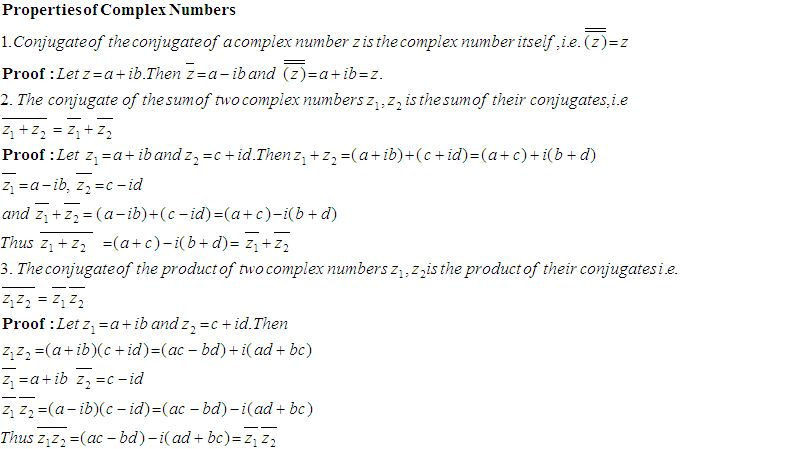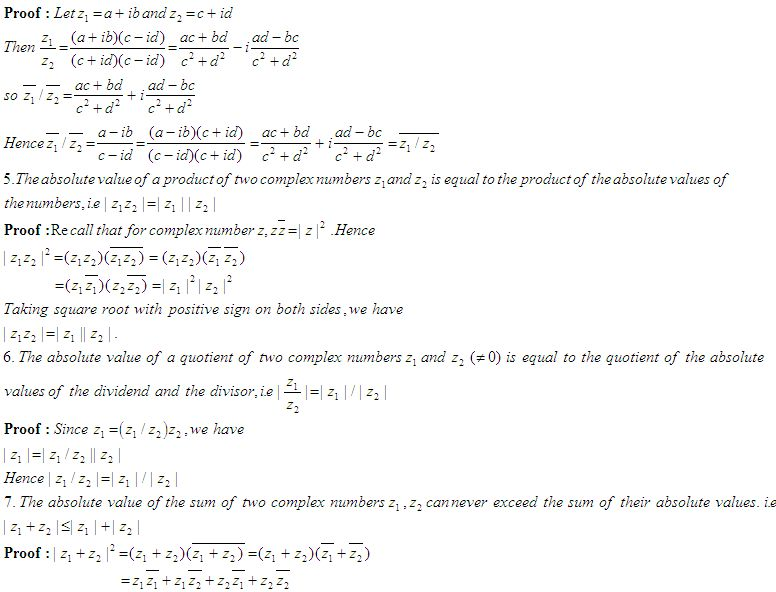Name: ___________________Date:___________________

 Email us to get an instant 20% discount on highly effective K-12 Math & English kwizNET Programs!

### High School Mathematics - 22.6 Some Properties of Complex NumbersDirections: Answer the following questions. Also write at least 5 examples of your own.
 Q 1: The conjugate of the product of two complex numbers z1 = 3+i7, z2 = 8+i57-i5735-i3321-i42 Q 2: Find the conjugate of the number 49-i7.Answer: Q 3: The conjugate of z1 + z2 given that z1 = 3+i5 and z2 = 6+i8 is ____.9-i139+i13-9-13i Q 4: Find x and y in (3y-2)+i(7-2x) = 0Answer: Q 5: Find x and y in 4x+i(3x-y) = 3-i6Answer: Q 6: If z = a+ib, what is the multiplicative inverse?a-ib1/a+ib1/a-ib Q 7: Find the conjugate of -iÖ5.Answer: Q 8: Find x and y in [3/(Ö5 x 5] + i2Ö5y = Ö2Answer: Question 9: This question is available to subscribers only! Question 10: This question is available to subscribers only!

#### Subscription to kwizNET Learning System costs less than \$1 per month & offers the following benefits:

• Unrestricted access to grade appropriate lessons, quizzes, & printable worksheets
• Instant scoring of online quizzes
• Progress tracking and award certificates to keep your student motivated
• Unlimited practice with auto-generated 'WIZ MATH' quizzes